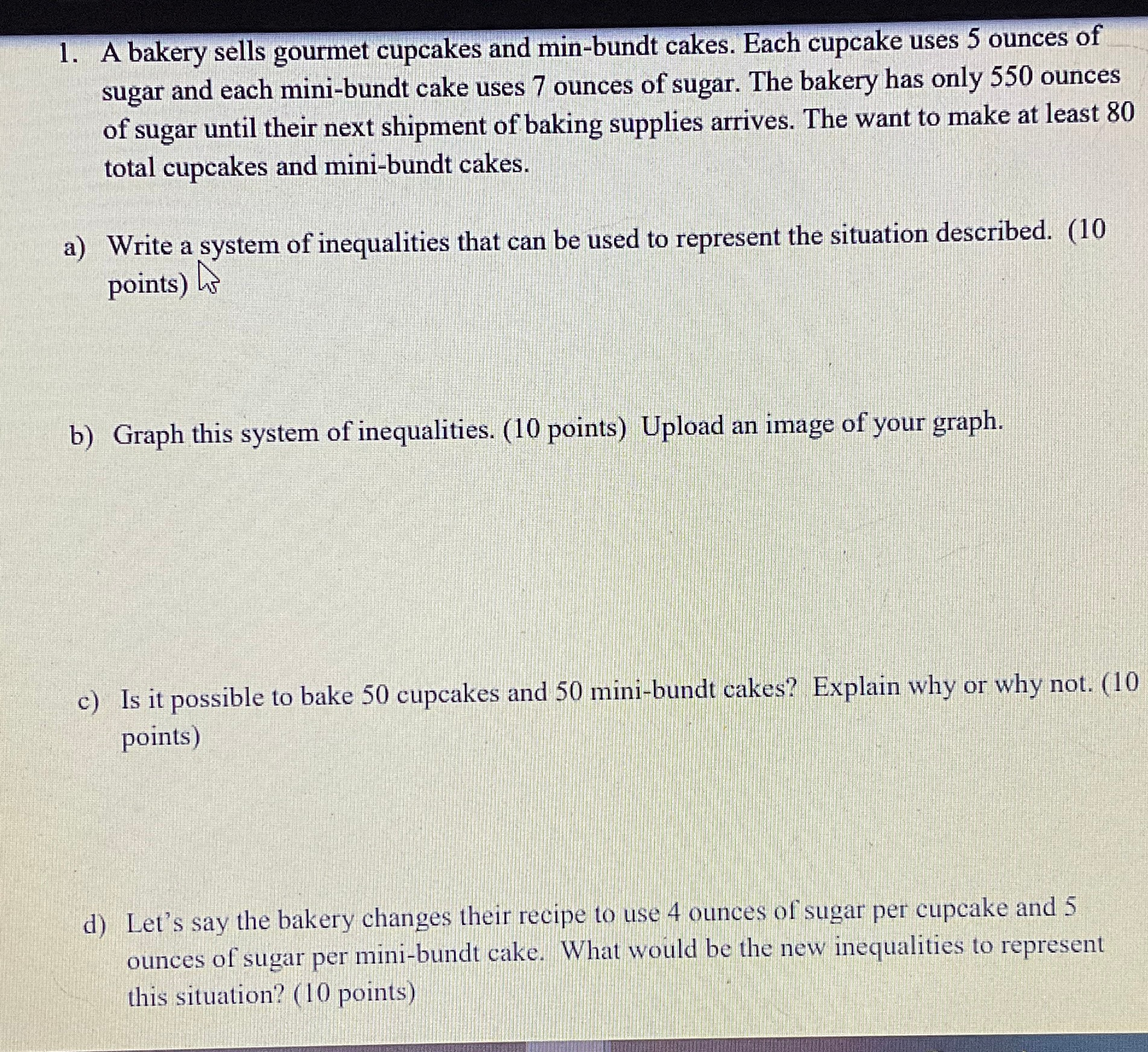### ¿Todavía tienes preguntas de matemáticas?

Pregunte a nuestros tutores expertos
Algebra
Pregunta1. A bakery sells gourmet cupcakes and min-bundt cakes. Each cupcake uses $$5$$ ounces sugar and each mini-bundt cake uses $$7$$ ounces of sugar. The bakery has only $$550$$ o of sugar until their next shipment of baking supplies arrives. The want to make at total cupcakes and mini-bundt cakes.

a) Write a system of inequalities that can be used to represent the situation described. points)

b) Graph this system of inequalities. (10 points) Upload an image of your graph.

c) Is it possible to bake $$50$$ cupcakes and $$50$$ mini-bundt cakes? Explain why or why points)

d) Let's say the bakery changes their recipe to use $$4$$ ounces of sugar per cupcake and ounces of sugar per mini-bundt cake. What would be the new inequalities to represent this situation? ( $$10$$ points)